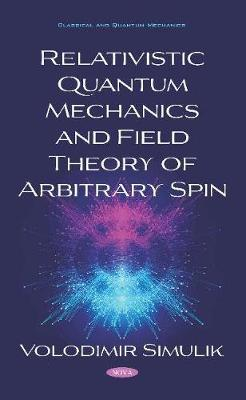•# Relativistic Quantum Mechanics and Field Theory of Arbitrary Spin (Hardback)

(author)
£163.99
Hardback 245 Pages / Published: 21/04/2020
• Coming soon

Awaiting publication

Foundations of the relativistic quantum mechanics and field theory of arbitrary spin are presented. New relativistic wave equations without redundant components for the particle-antiparticle doublets of arbitrary spin are considered. The comparison with known arbitrary spin equations of Bhabha, Bargman-Wigner and with Pauli-Fierz, Rarita-Schwinger equations (for the spin s=3/2) demonstrates the advantages of the presented approach. The special procedure of synthesis of higher spin relativistic wave equations is suggested. New equations are considered on three levels of (i) relativistic canonical quantum mechanics, (ii) canonical Foldy-Wouthuysen type field theory, and (iii) manifestly covariant field theory. The derivation of field equations based on the start from the relativistic canonical quantum mechanics is given. The corresponding transition operator, which is the extended Foldy-Wouthuysen transformation, is suggested and described. This model of relativistic quantum mechanics is described here on the level of von Neumann's consideration of non-relativistic case. The Lagrange approach for the spinor field in the Foldy-Wouthuysen representation is analyzed. The proof of the Fermi-Bose duality property of a few main equations of field theory, which before were known to have only single Fermi (or single Bose) property, is given. Hidden Bose properties (symmetry, solutions, and conservation laws) of the Dirac equation are proved. Both cases of non-zero and zero mass are considered. New useful mathematical objects, which are the pure matrix representations of the 64-dimensional Clifford and 28-dimensional SO(8) algebras over the field of real numbers, are put into consideration. The application of such algebras to the Dirac and Dirac-like equations properties analysis is demonstrated. Fermi and Bose SO(4) symmetries of the relativistic hydrogen atom are found. New symmetries and solutions of the Maxwell equations are considered. The Maxwell equations in the form, having maximal symmetry, are suggested and described. The application of such field-strength equations to the atomic microworld phenomena is demonstrated. On the basis of such Maxwell system the relativistic hydrogen atom spectrum and quantum properties of this atom are described. The Sommerfeld-Dirac fine structure formula, Plank constant and the Bohr postulates are derived in the frameworks of classical electrodynamics. The limits and boarders of classical physics applications in inneratomic microworld are discussed. In order to determine the place of our approach among other investigations the 26 variants of the Dirac equation derivation are considered.

Publisher: Nova Science Publishers Inc
ISBN: 9781536169874
Number of pages: 245